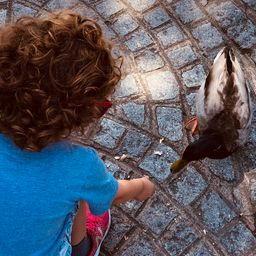Our Discord hit 10K members! 🎉 Meet students and ask top educators your questions.Join Here!Numerade Educator

# Statistics This table gives the mean temperature (in degrees Fahrenheit) for the month of January over a 20 -year period in Dickinson, ND.CAN'T COPY THE TABLE(a) Construct a box-and-whisker plot based on the data.(b) Discuss any significant features of the plot.(c) Compare the box-and-whisker plot constructed in exercise 39 with the one constructed in this exercise.

### Discussion

You must be signed in to discuss.
##### Top Geometry Educators##### Heather Z.

Oregon State University### Video Transcript

In order to make our box and whisker plot, we need our five number summary. Our minimum is negative. 2/10. Our first quartile is 10.6. Our median is 16.8. Our third court trial is 23.8 and we have a maximum of 27.7. Now that we have our five numbers, we can plot them on our number line. We have to start with negative too. So make sure there's room on your graph to go below zero. Then we have 10.6, 16.8, which is roughly here, 23.8 and then 27.7. Next drawn line through all of your five data points. Then connect your middle three in looking at this data, we see that we have one long route whisker to the left. We should probably check later on. If this number is an outlier, as negative two is notably further away than are right sided whisker the middle 50% of our data is roughly symmetrical, however, suggesting that 50% is clumped together overall in comparison to the previous graph. In the other problem, we have a much greater range. This is stretching over a higher volume of numbers. However, they both have roughly symmetrical centers, suggesting that the temperatures air fairly consistent for most of the data points. I would say, though, that this one has a more stretched out 50% than the other one, which had a smaller box. Both have extremely longer whiskers on some sides that suggests we should check for outliers.Boston University School of Medicine
##### Top Geometry Educators##### Heather Z.

Oregon State University